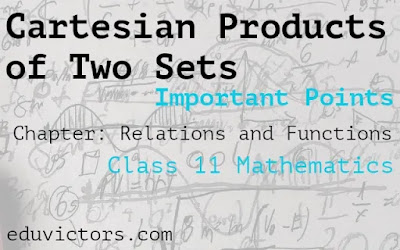Class 11 Maths - Cartesian Products of Two sets (Important Points)

Chapter: Relations and Functions

Let A and B be two nonempty sets. Then, the Cartesian product of A and B is the set denoted by (A×B), consisting of all ordered pairs (a, b) such that a ∈ A and b ∈ B.

∴ A × B = {(a, b): a ∈ A and b ∈ B }.

② If A = ϕ or B = ϕ (empty sets), we define A × B = ϕ

③ B × A = {(b, a) : b ∈ B and a ∈ A} and A × A = {(a,b):a,b ∈ A}.

If n(A) = p and n(B) = q, then

n(A×B)= n(A)⋅n(B)= pq.

A × (B ∪ C) = (A × B) ∪ (A × C)

A × (B ∩ C) = (A×B) ∩ (A×C)

A × (B − C) = (A × B) − (A × C)

(A × B) ∩ (C × D) = (A ∩ C) × (B ∩ D)

Arrow diagram of A × B

Let A = {-1, 2, 4} and B = {1, 3}, then

A × B = {(-1, 1), (-1, 3), (2, 1), (2, 3), (4, 1), (4, 3)}.

Then, A × B may be represented by arrow diagram, as shown below:

If A ⊆ B then  A × C ⊆ B × C for any set C.

If A ⊆ B and C ⊆ D then A × C ⊆ B × D.

If A and B are any two nonempty sets, A × B = B × A ⇔ A × B.

If A ⊆ B, A × A ⊆ (A×B) ∩ (B×A).

A ×(B ∪ C) = (A × B) ∪ (A × C).

A × (B ∩ C) = (A × B) ∩ (A × C).

A × (B - C) = (A × B) - (A × C).

(A × B) ∩ (C × D) = (A ∩ C) × (B ∩ D)

(A × B) ∩ (B × A) = (A ∩ B) × (B ∩ A).

Special Mathematical Constants

SETS (NCERT Ex 1.1)

Different Types of Sets

Ch2: Relations and Functions (1 Mark Q & A) Part-1